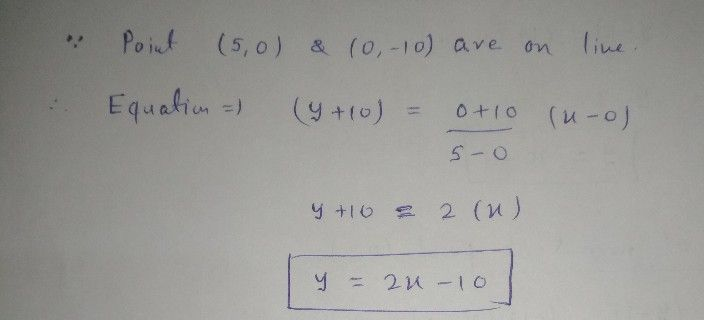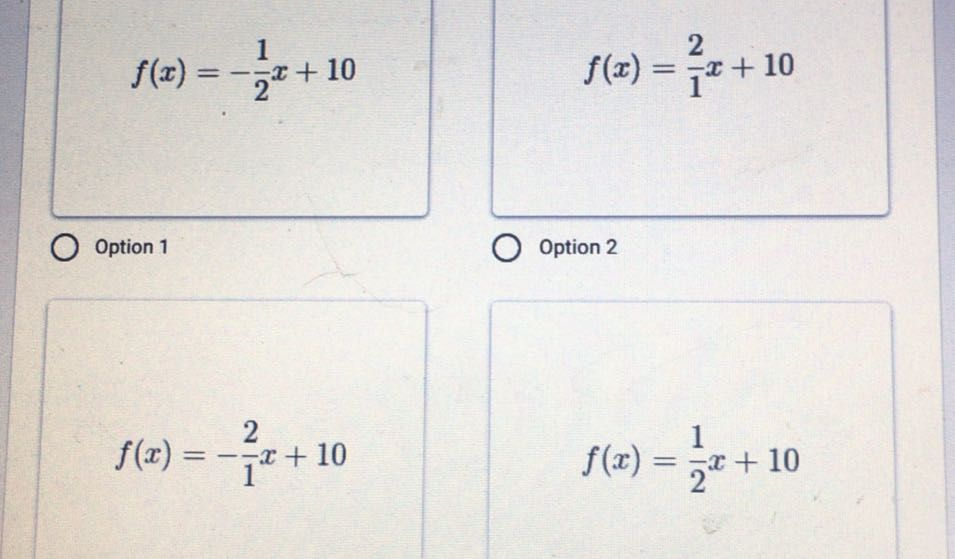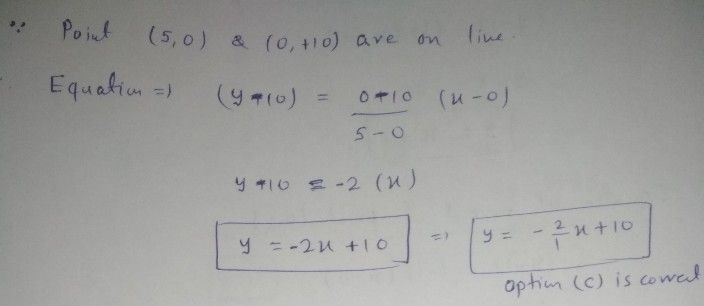Symbol
Problem$y$ $6$ $-10$ $-9$ $-8$ $p$ $-6$ $.5$ $6.5.a-B$ $k=1$ $1$ $6$ $89$ $10$ $X$ $2$ $3$ $-4$ $-6$ $-7$ $10$
Algebra
Question content
Write the equation for the following line
Solution